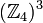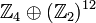# Group cohomology of groups of order 16

Jump to: navigation, search
This article gives specific information, namely, group cohomology, about a family of groups, namely: groups of order 16.
View group cohomology of group families | View group cohomology of groups of a particular order |View other specific information about groups of order 16
Group GAP ID second part Hall-Senior number If it is a finite group with periodic cohomology, then the period for the homology groups Group cohomology page
cyclic group:Z16 1 5 2 See group cohomology of finite cyclic groups
direct product of Z4 and Z4 2 3 -- group cohomology of direct product of Z4 and Z4; see also group cohomology of finite homocyclic groups
SmallGroup(16,3) 3 9 -- group cohomology of SmallGroup(16,3)
nontrivial semidirect product of Z4 and Z4 4 10 -- group cohomology of nontrivial semidirect product of Z4 and Z4
direct product of Z8 and Z2 5 4 -- See group cohomology of finite abelian groups
M16 6 11 -- group cohomology of M16
dihedral group:D16 7 12 -- group cohomology of dihedral group:D16; see also group cohomology of dihedral groups
semidihedral group:SD16 8 13 -- group cohomology of semidihedral group:SD16; see also group cohomology of semidihedral groups
generalized quaternion group:Q16 9 14 4 group cohomology of generalized quaternion group:Q16; see also group cohomology of dicyclic groups
direct product of Z4 and V4 10 2 -- See group cohomology of finite abelian groups
direct product of D8 and Z2 11 6 -- group cohomology of direct product of D8 and Z2
direct product of Q8 and Z2 12 7 -- group cohomology of direct product of Q8 and Z2
central product of D8 and Z4 13 8 -- group cohomology of central product of D8 and Z4
elementary abelian group:E16 14 1 -- group cohomology of elementary abelian group:E16, see also group cohomology of elementary abelian groups
View these in a broader context: group cohomology of groups of prime-fourth order | group cohomology of groups of order 2^n

## Homology groups for trivial group action

FACTS TO CHECK AGAINST (homology group for trivial group action):
First homology group: first homology group for trivial group action equals tensor product with abelianization
Second homology group: formula for second homology group for trivial group action in terms of Schur multiplier and abelianization|Hopf's formula for Schur multiplier
General: universal coefficients theorem for group homology|homology group for trivial group action commutes with direct product in second coordinate|Kunneth formula for group homology

### Over the integers

Group GAP ID second part Hall-Senior number Nilpotency class$H_1$ (= abelianization)$H_2$ (= Schur multiplier)$H_3$$H_4$$H_5$
cyclic group:Z16 1 5 1$\mathbb{Z}_{16}$ 0$\mathbb{Z}_{16}$ 0$\mathbb{Z}_{16}$
direct product of Z4 and Z4 2 3 1$\mathbb{Z}_4 \oplus \mathbb{Z}_4$$\mathbb{Z}_4$$(\mathbb{Z}_4)^3$$\mathbb{Z}_4 \oplus \mathbb{Z}_4$$(\mathbb{Z}_4)^4$
SmallGroup(16,3) 3 9 2$\mathbb{Z}_4 \oplus \mathbb{Z}_2$$\mathbb{Z}_2 \oplus \mathbb{Z}_2$$(\mathbb{Z}_4)^2 \oplus (\mathbb{Z}_2)^2$$(\mathbb{Z}_2)^5$$(\mathbb{Z}_4)^2 \oplus (\mathbb{Z}_2)^5$
nontrivial semidirect product of Z4 and Z4 4 10 2$\mathbb{Z}_4 \oplus \mathbb{Z}_2$$\mathbb{Z}_2$$(\mathbb{Z}_4)^2 \oplus \mathbb{Z}_2$$\mathbb{Z}_4 \oplus \mathbb{Z}_2$$(\mathbb{Z}_4)^2 \oplus (\mathbb{Z}_2)^2$
direct product of Z8 and Z2 5 4 1$\mathbb{Z}_8 \oplus \mathbb{Z}_2$$\mathbb{Z}_2$$\mathbb{Z}_8 \oplus (\mathbb{Z}_2)^2$$\mathbb{Z}_2 \oplus \mathbb{Z}_2$$\mathbb{Z}_8 \oplus (\mathbb{Z}_2)^3$
M16 6 11 2$\mathbb{Z}_4 \oplus \mathbb{Z}_2$ 0$\mathbb{Z}_8 \oplus \mathbb{Z}_2$$\mathbb{Z}_2$$\mathbb{Z}_4 \oplus (\mathbb{Z}_2)^2$
dihedral group:D16 7 12 3$\mathbb{Z}_2 \oplus \mathbb{Z}_2$$\mathbb{Z}_2$$\mathbb{Z}_8 \oplus (\mathbb{Z}_2)^2$$\mathbb{Z}_2 \oplus \mathbb{Z}_2$$(\mathbb{Z}_2)^4$
semidihedral group:SD16 8 13 3$\mathbb{Z}_2 \oplus \mathbb{Z}_2$ 0$\mathbb{Z}_8 \oplus \mathbb{Z}_2$$\mathbb{Z}_2$$(\mathbb{Z}_2)^3$
generalized quaternion group:Q16 9 14 3$\mathbb{Z}_2 \oplus \mathbb{Z}_2$ 0$\mathbb{Z}_{16}$ 0$\mathbb{Z}_2 \oplus \mathbb{Z}_2$
direct product of Z4 and V4 10 2 1$\mathbb{Z}_4 \oplus (\mathbb{Z}_2)^2$$(\mathbb{Z}_2)^3$$\mathbb{Z}_4 \oplus (\mathbb{Z}_2)^6$$(\mathbb{Z}_2)^8$$\mathbb{Z}_4 \oplus (\mathbb{Z}_2)^{12}$
direct product of D8 and Z2 11 6 2$(\mathbb{Z}_2)^3$$(\mathbb{Z}_2)^3$$\mathbb{Z}_4 \oplus (\mathbb{Z}_2)^6$$(\mathbb{Z}_2)^8$$(\mathbb{Z}_2)^{13}$
direct product of Q8 and Z2 12 7 2$(\mathbb{Z}_2)^3$$(\mathbb{Z}_2)^2$$\mathbb{Z}_8 \oplus (\mathbb{Z}_2)^3$$(\mathbb{Z}_2)^3$$(\mathbb{Z}_2)^6$
central product of D8 and Z4 13 8 2$(\mathbb{Z}_2)^3$$(\mathbb{Z}_2)^2$$\mathbb{Z}_8 \oplus (\mathbb{Z}_2)^3$$(\mathbb{Z}_2)^3$$(\mathbb{Z}_2)^6$
elementary abelian group:E16 14 1 1$(\mathbb{Z}_2)^4$$(\mathbb{Z}_2)^6$$(\mathbb{Z}_2)^{14}$$(\mathbb{Z}_2)^{21}$$(\mathbb{Z}_2)^{35}$

## Second cohomology groups and extensions

### Schur multiplier and Schur covering groups

FACTS TO CHECK AGAINST for Schur multiplier of group of prime power order
ABELIAN CASE: Schur multiplier of finite abelian group is its exterior square
UPPER BOUNDS: upper bound on size of Schur multiplier for group of prime power order based on prime-base logarithm of order |upper bound on size of Schur multiplier for group of prime power order based on prime-base logarithm of order and exponent of center
LOWER BOUNDS: lower bound on size of Schur multiplier for group of prime power order based on minimum size of generating set
Group GAP ID 2nd part Hall-Senior number Nilpotency class$H_2$ (= Schur multiplier) Order of$H_2$ Possibilities for Schur covering groups Cohomology group information Orders of Schur covering groups exterior square, which is the derived subgroup of every Schur covering group (note that if the original group is abelian, this coincides with the Schur multiplier; in general, its order is that of the Schur multiplier times that of the derived subgroup of the starting group) quotient group by epicenter, which is the inner automorphism group of every Schur covering group (note that if the original group is a capable group, it is equal to the original group; in the abelian case, see characterization of epicenter of finite abelian group)
cyclic group:Z16 1 5 1 trivial group 1 cyclic group:Z16 -- 16 trivial group trivial group
direct product of Z4 and Z4 2 3 1 cyclic group:Z4 4 unitriangular matrix group:UT(3,Z4), SmallGroup(64,19), and semidirect product of Z16 and Z4 via fifth power map second cohomology group for trivial group action of direct product of Z4 and Z4 on Z4 64 cyclic group:Z4 direct product of Z4 and Z4
SmallGroup(16,3) 3 9 2 Klein four-group 4 SmallGroup(64,8), possibly others second cohomology group for trivial group action of SmallGroup(16,3) on Klein four-group 64 direct product of Z4 and Z2 SmallGroup(16,3)
nontrivial semidirect product of Z4 and Z4 4 10 2 cyclic group:Z2 2 semidirect product of Z8 and Z4 of dihedral type, possibly others. second cohomology group for trivial group action of nontrivial semidirect product of Z4 and Z4 on Z2 32 cyclic group:Z4 dihedral group:D8
direct product of Z8 and Z2 5 4 1 cyclic group:Z2 2 SmallGroup(32,5), possibly others. second cohomology group for trivial group action of direct product of Z8 and Z2 on Z2 32 cyclic group:Z2 Klein four-group
M16 6 11 2 trivial group 1 M16 -- 16 cyclic group:Z2 Klein four-group
dihedral group:D16 7 12 3 cyclic group:Z2 2 dihedral group:D32, generalized quaternion group:Q32, semidihedral group:SD32 second cohomology group for trivial group action of D16 on Z2 32 cyclic group:Z8 dihedral group:D16
semidihedral group:SD16 8 13 3 trivial group 1 semidihedral group:SD16 -- 16 cyclic group:Z4 dihedral group:D8
generalized quaternion group:Q16 9 14 3 trivial group 1 generalized quaternion group:Q16 -- 16 cyclic group:Z4 dihedral group:D8
direct product of Z4 and V4 10 2 1 elementary abelian group:E8 8 SmallGroup(128,170) and possibly others  ? 128 elementary abelian group:E8 elementary abelian group:E8
direct product of D8 and Z2 11 6 2 elementary abelian group:E8 8 SmallGroup(128,731) and possibly others  ? 128 direct product of Z4 and V4 direct product of D8 and Z2
direct product of Q8 and Z2 12 7 2 Klein four-group 4 SmallGroup(64,74) and possibly others second cohomology group for trivial group action of direct product of Q8 and Z2 on V4 64 elementary abelian group:E8 elementary abelian group:E8
central product of D8 and Z4 13 8 2 Klein four-group 4 SmallGroup(64,75) and possibly others  ? 64 elementary abelian group:E8 elementary abelian group:E8
elementary abelian group:E16 14 1 1 elementary abelian group:E64 64  ?  ? 1024 elementary abelian group:E64 elementary abelian group:E16